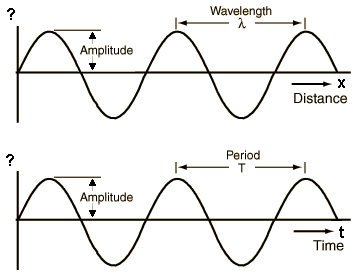Deutsche Version● Wave Graphs ●
Amplitude, Distance and Time

 Waves may be graphed as a function of time or distance. A single frequency wave will appear as a sine wave (sinusoid) in either case. From the distance graph the wavelength may be determined. From the time graph, the period and the frequency can be obtained. From both together, the wave speed can be determined. Frequency is cycles per second. Source: http://hyperphysics.phy-astr.gsu.edu/hbase/sound/wavplt.htmlAny of the wave parameters below can be changed. When you have finished entering data, click on the quantity you wish to calculate. The quantities will not be forced to be consistent until you click on the desired quantity.

 Wavelength λ m Frequency f Hz Period T s Amplitude a 2 Wave velocity ν m/s
 Physical value symbol unit abbreviation formula period duration T = 1 / f second s T = λ / ν Frequency f = 1 / T hertz Hz = 1/s f = ν / λ Wavelength λ meter m λ = ν / f Wave speed ν meter per second m/s ν = λ × f Speed of sound c meter per second m/s c = λ × f

 Amplitude as particle displacement ξ = p / (2 π × Z) Ampliude as sound pressure p = ξ ×2 π × Z = v × Z Specific acoustic impedance of air at 20°C is Z = 413 N·s/m3 Speed of sound of air at 20°C is c = 343 m/s   "Distance = velocity × time" is the key to the basic wave relationship.

 The change of audio frequency does not change the amplitude and vice versa.